Reach Us+44-1522-440391
Bayesian Corrections of a Selection Bias in Genetics | OMICS International
Journal of Biometrics & Biostatistics

Like us on:

All submissions of the EM system will be redirected to Online Manuscript Submission System. Authors are requested to submit articles directly to Online Manuscript Submission System of respective journal.

# Bayesian Corrections of a Selection Bias in Genetics

Balgobin Nandram1 and Hongyan Xu2*

1Department of Mathematical Sciences, Worcester Polytechnic Institute, Worcester, MA

2Department of Biostatistics, Medical College of Georgia, Augusta, GA

*Corresponding Author:
Hongyan Xu, PhD
Department of Biostatistics
Medical College of Georgia
1120 15th St., Augusta, GA 30912
Tel: (706) 721-3785
Fax: (706) 721-6294
E-mail: [email protected]

Received date: November 17, 2010; Accepted date: March 7, 2011; Published date: March 10, 2011

Citation: Nandram B, Xu H (2011) Bayesian Corrections of a Selection Bias in Genetics. J Biomet Biostat 2:112. doi: 10.4172/2155-6180.1000112

Copyright: © 2011 Nandram B, et al. This is an open-access article distributed under the terms of the Creative Commons Attribution License, which permits unrestricted use, distribution, and reproduction in any medium, provided the original author and source are credited.

Visit for more related articles at Journal of Biometrics & Biostatistics

#### Abstract

When there is a rare disease in a population, it is inefficient to take a random sample to estimate a parameter. Instead one takes a random sample of all nuclear families with the disease by ascertaining at least one sibling (proband) of each family. In these studies, if the ascertainment bias is ignored, an estimate of the proportion of siblings with the disease will be inflated. The problem arises in population genetics, and it is analogous to the well-known selection bias problem in survey sampling. For example, studies of the issue of whether a rare disease shows an autosomal recessive pattern of inheritance, where the Mendelian segregation ratios are of interest, have been investigated for several decades and corrections have been made for the ascertainment bias using maximum likelihood estimation. Here, we develop a Bayesian analysis to estimate the segregation ratio in nuclear families when there is an ascertainment bias. We consider the situation in which the proband probabilities are allowed to vary with the number of affected siblings, and we investigate the effect of familial correlation among siblings within the same family. We discuss an example on cystic fibrosis and a simulation study to assess the effect of the familial correlation.

#### Keywords

Familial correlation; Monte carlo methods; Population genetics; Segregation ratio; Truncated binomial distribution

#### Introduction

When there is a rare disease in a population, it is inefficient to take a random sample to estimate a parameter. Instead one takes a random sample of all nuclear families with the disease by ascertaining at least one sibling (proband) of each family. In these studies, an estimate of the proportion of siblings with the disease will be inflated. Sometimes the situation is even worse; the investigator takes all the families that appear. Thus, there is a selection bias .

Fisher  illustrated the importance of adjusting for the selection bias in genetics; see also  for a discussion of ascertainment bias in the analysis of family data. For example, studies of the issue of whether a rare disease shows an autosomal recessive (dominant) pattern of inheritance, where the Mendelian segregation ratios are of interest, have been investigated for several decades. The Mendelian segregation ratio is p = 0.5 for an autosomal dominant disease and p = .25 for an autosomal recessive disease. These follow from the first law of Mendel. For a rare disease one would be interested to know whether it is autosomal dominant or recessive. That is, whether p = 0.5 or p = .25 respectively. But because the disease is rare, the investigator will select all those nuclear families that appear. Then there is a selection bias; specifically the estimates will be inflated. See also chapter 2 of  and chapter 2 of  for very clear pedagogy on this problem. How do we correct for this ascertainment bias? Non-Bayesian methods are available to correct for the ascertainment bias. Specifically, see  for a review and a discussion of difficulties associated with maximum likelihood estimation for the ascertainment bias problem.

Here, we develop a Bayesian analysis to estimate the segregation ratio in nuclear families when there is an ascertainment bias. To our knowledge this is the first Bayesian approach to the ascertainment bias problem in genetics. More importantly, we investigate the effects of familial correlation among siblings within the same family. It is expected that one sibling getting affected will be related to the other siblings because they are in the same nuclear family sharing the same genes. In addition, we investigate the effects of heterogeneous familial correlations and proband probabilities. Again, these analyses are new within the Bayesian paradigm, and there has not been any frequentist analysis with heterogeneity. The Bayesian analysis is useful because we can obtain exact distributions under the specified model, and we can input important prior information (e.g., about the genetic features of cystic fibrosis).

Cystic fibrosis is a hereditary disease that affects the mucus glands of the lungs, liver, pancreas, and intestines, causing progressive disability due to multisystem failure. The CFTR gene, found in Chromosome 7, is the cause of cystic fibrosis, where mutations result in proteins that are too short because of premature end to production. We have been analyzing data on cystic fibrosis for the School of Medicine, Medical College of Georgia, and because of confidentiality issues we cannot present these data in this paper. Although these data are very sparse with only a few individuals reported cystic fibrosis in southern Georgia, our data set has the same structure as one that has been used repeatedly in the literature.

Table 1 gives a set of data on cystic fibrosis, which was presented by Crow  to illustrate the need to take account of the method of ascertainment in segregation analysis. One can countthe total number of offspring to be 269, the total number of affected offspring to be 124, and the total number of probands to be 90. Thus, one might estimate the segregation ratio to be 124/269 = .4610, and the ascertainment probability to be 90/124 = .7258. Again, these simple estimates are too inflated. Note that 46.1% is far in excess of the 25% expected on simple recessive inheritance (cystic fibrosis is autosomal recessive). One reason for the excess is the ascertainment bias - the exclusion of families where the parents are heterozygous, but fail to have a homozygous recessive child. These would add to the number of normal children and thereby reduce the proportion affected. This data set was also used in  for illustration.

Size Affected Proband Families
10 3 1 1
9 3 1 1
8 4 1 1
7 3 2 1
7 3 1 1
7 2 1 1
7 1 1 1
6 2 1 1
6 1 1 1
5 3 3 1
5 3 2 1
5 2 1 5
5 1 1 2
4 3 2 1
4 3 1 2
4 2 1 4
4 1 1 6
3 2 2 3
3 2 1 3
3 1 1 10
2 2 2 2
2 2 1 4
2 1 1 18
1 1 1 9

Table 1: Number of families affected by sibship size, number affected offspring and number of probands (Crow 1965).

When all families with affected offspring are ascertained, we say that there is complete ascertainment; otherwise there is incomplete ascertainment and in this case (unknown to the investigator) there are families with affected siblings who are not probands. When there is complete ascertainment, the proband probability is one; otherwise it is distinctly less than one. Fisher  first analyzed the data using complete ascertainment. His analysis was done using a truncated Binomial distribution. However, Fisher  also described a simpler method for the more appropriate incomplete ascertainment for these data. This discussion was further developed by Bailey  and Morton . In this paper, we will focus on incomplete ascertainment as is evident in Table 1. Crow  pointed out for the cystic fibrosis data the need to adjust for ascertainment bias and incomplete ascertainment.

The key idea for the correction of ascertainment bias is to find the correct sampling distribution under the ascertainment bias. Let x represent the quantity being measured, and let A denote the ascertainment event. Without the ascertainment bias, f(x|θ) is the sampling distribution for a random sample. This is an example of an ignorable selection model. However, when there is an ascertainment bias, we need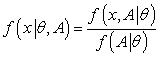That is, we condition on the ascertainment event, A. Here f(x| θ, A) provides a non- ignorable selection model. In general, the two sampling distributions f(x| θ, A) and f(x| θ) are different; f(x| θ, A) is the more appropriate sampling distribution. Correcting for ascertainment bias means that we need to construct the sampling distribution, f(x| θ, A). A simple example, introduced in  for complete ascertainment, is on the number r siblings affected in a family of size s in a binomial model with r> 0. Then,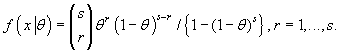Here, A is the event that r> 0, leading to the binomial distribution truncated at 0. More importantly the binomial probabilities are being re-weighted so that all the mass points are 1, . . . , s. That is, assuming that each sib- ling is affected independently, then P (r> 0 |θ) = 1 - P (none of the s siblings is affected) =1 - (1 - θ)s.

The problem of ascertainment bias is not new to survey samplers. For finite population sampling, Sverchkov and Pfeffermann  defined the sample and sample-complement dis- tributions as two separate weighted distributions (see ) for developing design consistent predictors of the finite population total; see also the more recent presentation . Malec et al.  used a hierarchical Bayesian method to estimate a finite population mean whenthere are binary data. These works are not directly applicable to our situation, but the ideas they portray are important for the issues associated with ascertainment bias. For probability proportional to size (PPS) sampling, Nandram  used surrogate sampling techniques to provide simulated random samples by using a model which reverses the selection bias. Under PPS sampling, Nandram et al.  used a method, developed by , to perform Bayesian predictive inference when a transformation is needed.

We distinguish between two ascertainment bias problems in population genetics. One occurs in the study of rare Mendelian disorders, and the other in single nucleotide polymorphism discovery.

We describe the first ascertainment bias problem. It is almost the case that a disease is inherited from recessive parents when the disease is rare in the entire population. The number of at-risk parents is usually small (i.e., the number of parents capable of producing affected siblings is very small relative to the number not capable of producing affected siblings). So if a sample is taken at random from the entire population, there could be no at-risk families. Thus, at-risk families are divided into two groups, those with at least one affected sibling and the other with no affected siblings. A sample is then drawn from the families with at least one affected sibling, thereby introducing an ascertainment bias. Thus, a direct estimate of the proportion of affected siblings will be too large; one needs to adjust for the ascertainment bias. Our example on cystic fibrosis falls in this first category of ascertainment bias problems.

We describe the second ascertainment bias problem. The human genome has very low density of polymorphisms, and the single nucleotide polymorphism (SNP) discovery has an ascertainment bias. The strategy of using a small sample (panel) followed by genotyping of a large sample in SNP discovery saves time and money. In SNP discovery a small sample of people is taken from the population, and these individuals are genotyped for a large number (≈ 106) of nucleotides. However, because of the low density of polymorphisms, many of the nucleotides of the panel are not polymorphic, and they are eliminated from the panel (i.e., they are not variable in the panel). The discovery goes on to genotyping a larger sample for the variable nucleotides (i.e., the remaining nucleotides). But, if the panel sample was larger, some of the discarded nucleotides could have been polymorphic in the population. Thus, there is an ascertainment bias. Kuhner et al.  show that representing panel SNPs as sample SNPs leads to large errors in estimating population parameters. Their recommendation to collect and preserve information about the method of ascertainment is very sensible. Clark et al.  point out that ascertainment bias will likely erode the power of tests of association between SNPs and complex disorders. Nielsen and Signorovitch  review some of the current methods of SNP discovery, and derive sample distributions of single SNPs and pairs of SNPs for some common SNP discovery schemes. They also show that the ascertainment bias in SNP discovery has a large effect on the estimation of linkage disequilibrium and recombination rates, and they describe some methods of correcting for ascertainment biases when estimating recombination ratios from SNP data.

In this paper we provide a Bayesian analysis of the ascertainment bias problem in which we assume incomplete ascertainment for rare recessive disease, not the SNP problem. The plan of the rest of the paper is as follows. In basic models, theory and estimation section, we present the basic models, theory, estimation, and a Bayesian analogue of the existing method. In Bayesian analysis with familial correlation section, we discuss the issue of incorporating a familial correlation in the ascertainment model, and we provide a simulation study to assess the effect of the ascertainment bias and the familial correlation. In heterogeneous probabilities and correlations section, we investigate the effect of heterogeneous proband probabilities and familial correlations using the cystic fibrosis data. In conclusion section, we provide concluding remarks, and we discuss ascertainment bias in SNP discovery.

#### Basic Models, Theory and Estimation

Thompson  discussed many ascertainment models. In this paper, we discuss the simplest ascertainment model  and . Essentially Lange  shows how to adjust for the ascertainment bias using the EM algorithm ; Sham  uses Fisher's scoring. In basic selection models section, we describe the basic selection models, ignorable and nonignorable, and in Properties of the joint probability mass function section, we describe some properties of the joint probability mass function for the nonignorable selection model. In bayesian method section, we present a simple Bayesian method of the ascertainment bias problem.

Basic selection models

Suppose there are n families selected through ascertainment sampling. Letting the kth ascertained family have sk siblings, we assume that there are rk affected and ak ascertained. In Crow's data sk vary from 1 to 10. The simplest ascertainment model specifies that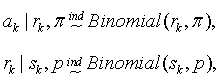k = 1, . . . , n. This is the basic ignorable selection model. The ak are really covariates, and this leads to improved precision. Thus, the joint probability mass function of (ak, rk) is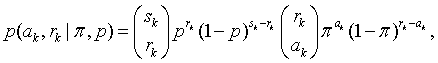(1)

ak= 0,...,rk, rk= 0,...,sk, k= 1,...,n. Note that (1) provides the likelihood for any family without conditioning on whether it is ascertained or not. To adjust for ascertainment bias, we need to restrict (1) to the support 1≤ak≤rk≤sk,k=1,...,n. This adjustment of the basic ignorable selection model gives the basic nonignorable selection model.

The probability that a family with sk siblings is ascertained is 1− (1− pπ )sk. This is the probability that there is at least one affected sibling (i.e., at least one proband is identified). This leads to the truncated probability mass function for the basic nonignorable selection model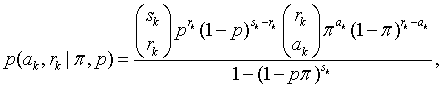(2)

ak=1,..., rk, rk=ak,...,sk. Note that (2) provides the likelihood for a family that has been ascertained. Thus, in the terminology of missing data, while (1) is the complete data likelihood, (2) is the sincomplete data likelihood. Note that in (2) 1- (1- pp )sk is simply the probability that 1≤ak≤rk≤sk, k=1,...,n. Thus, p(ak, rk|p, p) actually includes the ascertainment event in the condition; henceforth, it is convenient to omit this conditioning.

Now a reasonable assumption is that the families are sampled independently. Then the likelihood function for all ascertained families is

Likelihood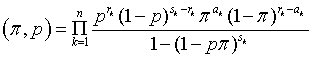(3)

The logarithm of the likelihood function of (Π, p) in (3) can be maximized, and one can use a normal approximation for the joint distribution of the maximum likelihood estimators. Sham  used the method of scoring, and Lange  used the expectation maximization (EM) algorithm. Nandram et al.  described three other algorithms: Newton's method, the Nelder-Mead algorithm and a new simple iterative algorithm. For example, for Crow's data, the EM algorithm gives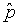= .268,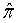= .359; the standard errors are respectively .0347 and .0814 with a small correlation of .248. These are consistent with the estimates given by Lange  and the algorithms of ; Lange  did not present the standard errors. As pointed by , these estimates are consistent with the theoretical value of 5 .25 for an autosomal recessive as in cystic fibrosis.

Properties of the joint probability mass function

We describe some useful properties and interpretations of the joint probability mass function in (2).

Using (2), the marginal probability mass function of rk is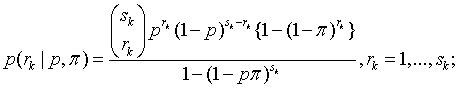All other points have zero probability. (This is obtained by simply summing over ak.) By using the probability mass function, p(rk|p, Π), one can show that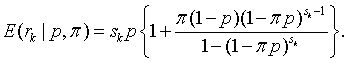(4)

Thus, E(rk |p, Π)is bigger than skp with the discrepancy related to p, Π and sk. With some cumbersome algebraic manipulation, it can be shown that

Var(rk|p, Π)=skp(1-p)(1-Qk),

where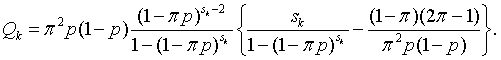Note Qk≤1 (i.e., Qk is an adjustment factor). So that if Qk≥0, then Var(rk|p, Π)≤skp (1-p), the situation in which rk|p~Binomial (sk, p). For example, if sk= 1, then Qk = {1-Π p-2Π (1-Π)}/ Πp (1-Πp). If, in addition, (reasonable for autosomal recessive), then 0 ≤Qk=1 and Var(rk|p, Π) ≤ p (1-p).

Also, for a family that has not been ascertained (i.e., ak = 0), it is easy to show that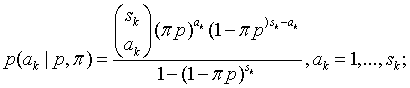Here, (1-Π p)sk -{(1-Π ) psk is the probability of having at least one affected sibling in the kth family with ak= 0.

The marginal probability mass function of ak is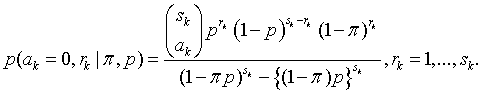All other points have zero probability. It is easy to show that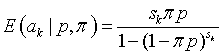(5)

and

Var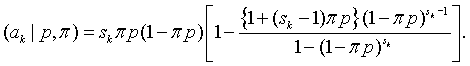Thus, as expected, E(ak| p, Π) increases from sk Πp, and Var(ak | p, Π) decreases from sk Π p(1-Π p).

We can also show that the correlation between ak and rk is nonnegative as follows. It is easy to show that

Cov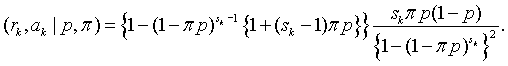But because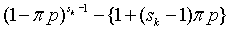is a nonnegative decreasing function of sk starting at sk= 1 with the value of 1, the correlation must be nonnegative.

The conditional probability mass function of rk given ak is also interesting. It is easy to show that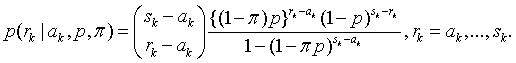That is, rk-ak |ak, Π, p~Binomial{sk-ak,(1- Π)p/(1- Π p)}. Then

and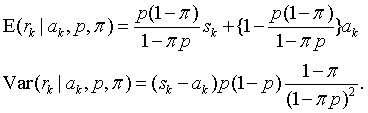Thus, in the conditional probability mass function, expectation increases with ak and variance decreases with ak. That is, knowledge of ak is informative, consistent with . Sham  used data from  to illustrate this issue, but here we have obtained an analytical argument.

Bayesian method

We consider Bayesian inference about p and Π in which (3) is the likelihood function. This is accomplished by using the noniformative proper priors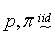Uniform(0,1).

Then, using Bayes’ theorem, the joint posterior density of (π, p) is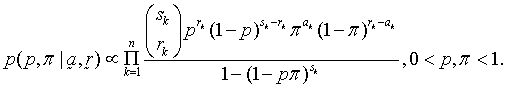(6)

Note that the uniform is updated using the likelihood (3) to get the joint posterior density in (6). Also, note that it is the term, 1- (1- pΠ )sk , in the denominator of (6) which primarily contributes to the complexity of the two-dimensional posterior density.

To make posterior inference about (p, Π), one can use standard numerical integration. However, it is simpler and more convenient to draw a random sample from the joint posterior density. Of course, one can use a Metropolis sampler to fit (draw a sample) from (6). This requires monitoring of convergence and it provides dependent samples. It is much simpler and more elegant to draw a sample from (6) using a grid method because the posterior density lies in the unit square, and it is easy to calculate. Thus, in this case we do not need to use Markov chain Monte Carlo methods.

To draw the bivariate sample of the posterior density of (p, Π), we use a grid method in the unit square (0, 1) by (0, 1), the full domain of the joint posterior density (p, Π) in (6). Our method allows us to construct a discrete bivariate approximation to the joint posterior density. We divide the interval (0, 1) into 100 intervals; so there are 10,000 little squares in the original unit square. We obtain the heights of the posterior density (without the normalization constant) at the center of each of the 10,000 squares. Because these little squares have the same area, the heights of the bivariate density are proportional to the posterior probabilities that (p, Π) fall in each of these squares. Thus, we have constructed a joint posterior mass function of (p, Π) on very fine grids. It is easy to draw a sample from the discrete bivariate probability mass function by using the cumulative distribution method. Each draws gives us one of the 10, 000 little squares, and then a random jittering is performed in the selected square. This is actually a random draw of one of the 10,000 squares with probabilities proportional to the heights of the little squares. Then within the selected square we choose a point at random by drawing two uniform random variables (i.e., uniform random jittering). This is a very accurate random draw from the joint posterior density in (6). We draw M = 10, 000 samples from this approximation for posterior inference in a standard Monte Carlo procedure with independent samples, not a Markov chain. Because of the random jittering the numbers are different with probability one. This goes at the blink of an eye! For example, letting (p(h), Π (h)), h = 1, . . . , M, denote the probability sample of size M from the bivariate distribution, then for any function H (p, Π) we can obtain the posterior mean as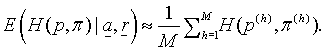While our grid method is similar to the method of Gelman, Carlin, Stern and Rubin, 2004 , there is one important difference. We know that the domain of the joint posterior density is in (0, 1) 2, the unit square, and for all practical purposes (p, Π) are not on the boundary of the parameter space. Also, we can explore the entire domain using small grids of dimension .012. Thus, unlike , we do not need to search for the 'modal region' of the posterior density. Moreover, the posterior density (without the normalization constant) is easy to calculate. In fact, our procedure is an improvement over the grid method described in .

For Crow's data the posterior mean, posterior standard deviation and the 95% credible intervals for p are .271, .035, (.206, .340) and for ? are .364, .079 and (.210, .513). Note that the 95% credible interval for p contains .250, consistent with an autosomal recessive.

Bayesian Analysis with Familial Correlation

We investigate the effect of familial correlation among siblings within the same family. We start by adding an intra-class correlation to the model with a single proband probability. One can expect an intra-class correlation because siblings of the same nuclear family are genetically similar to some degree. For example, one sibling getting cystic fibrosis will be related to another getting infected because they have some common genes. Our new model contains a nonnegative intra-class correlation θ similar to ; see also  for developments in two-way categorical tables and the effects of intra-class correlation to the chi-square test. We will also describe a model that does not incorporate any information about the ascertainment bias; this is the ignorable selection model. The model that incorporates the selection bias will be called the nonignorable selection model.

Sampling distributions

First, we describe the sampling distribution, p(rk,ak |p, Π, θ), where θ is the intra-class (familial) correlation. With sk siblings in the kth family, using a formula of , we have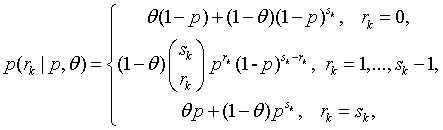where k=1,...,n, 0 ≤ θ ≤ 1. Note that when sk=1 there are only three possible values of (ak, rk) with positive probabilities; these are (0,0), (0,1) and (1,1). w

When θ=0, we get the original model, and when θ=1, we get p(rk=sk|p, θ) = p=1-p(rk= 0 |p, θ) with p(rk|p, θ)= 0, rk= 0,..., sk-1. With a perfect correlation, in a family there is effectively only one observation. Note that E(rk| p, θ) = skp and var(rk |p, θ) = skp(1-p){1+(sk-1) θ}. Thus, the intra-class correlation increases the variance, but it keeps the mean unchanged.

It is useful to note that for rk = 1, . . . ,sk,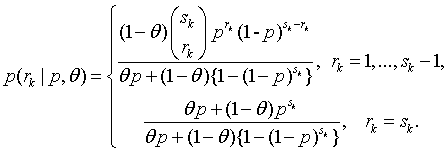Then, it is easy to show that E(rk|p,θ)=Skp[1+w1(1-p)/p+(1-w1) (1-p)sk /{1-(1-p)sk }], where w1=θp/[θp+(1-θ{1-(1-p)sk }]. Thus, when θ=0, w1=0 and E(rk |p, θ)= sk p(1-p)sk /{1-(1-p) }; and when θ =1, w1=1 and, as expected, E(rk|p,θ)=sk. Here (1- p)/p is the odds for no affected sibling in a family, and (1-p)sk /{1-(1-p)sk } is the odds of at least one affected sibling.

In Appendix A, we show how to obtain the joint probability mass function of (ak, rk) for ascertained families, 1≤ak ≤rk ≤sk. For rk =1,...,sk-1,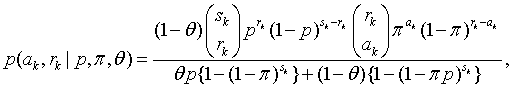and for rk= sk,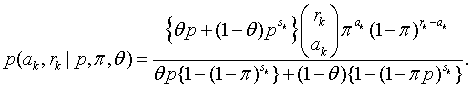This is the nonignorable selection model (i.e., the model that accommodates the ascertainment bias). In Appendix A, we also show that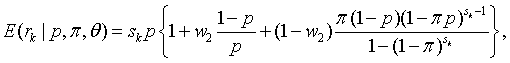where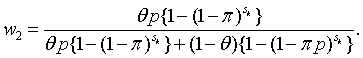Note that when sk=1under ascertainment bias ak=rk =1 with probability one; so all families with exactly one sibling are excluded from the analysis.

For comparison, we briefly describe the ignorable selection model. Essentially this is the model without the normalization constant in (ak, rk|p, Π, θ) (i.e.,0=ak=rk=sk). It is useful to separate the probability mass function of (ak,rk) into the following four parts. For 0≤ak ≤rk ≤1,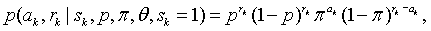and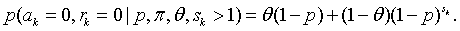For rk = 1, . . . , sk-1,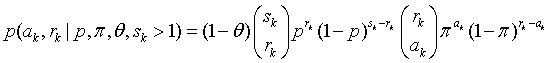and for rk = sk,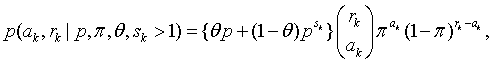where ak = 0, . . . , rk .

Posterior inference

We use the same assumption as in the original model that (ak,rk) are independent over families (k=1, . . . ,n), and we assume thatUniform(0,1) Then, using Bayes' theorem, the joint posterior density of (p, Π, θ) is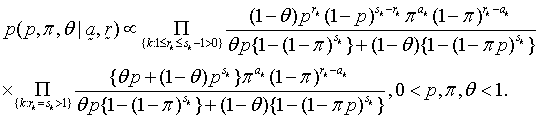(7)

For the ignorable selection model, the joint posterior density is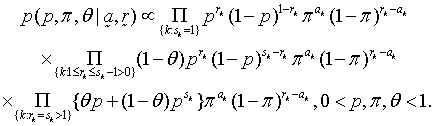(8)

Note that in (8) there is no term with ak=rk=0 because they are simply not in the data of ascertained families.

To make posterior inference about (p, Π, θ), we use a grid method in three dimensions in a manner similar to the one discussed earlier for (p, Π). With 100 intervals in each variable, we have to evaluate the joint posterior density at 106 values of (p, Π, θ), not too time-consuming though. It is unnecessarily complex to run a Gibbs sampler here. Because each of p, Π and θ lives in (0, 1), the grid procedure is still attractive. Note that for the ignorable selection model, a posteriori p and θ are jointly independent of Π. In fact,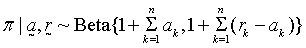and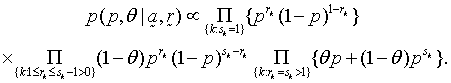Thus, we use a grid to draw (p, Π), and we draw Π independently. In either case, we have used 10, 000 iterations, perhaps too many!

In Table 2 we have compared the ignorable and the nonignorable selection models for Crow's data when inference is made for p, Π and θ. The correlation is almost zero under both the ignorable and the nonignorable selection models, but the difference between these models for inference about p and Π is enormous with much larger estimates from the ignorable selection model. Under the nonignorable selection model, the posterior mean, posterior standard deviation and 95% credible interval for p are .257, .033, (.190, .320). This small correlation seems to have some effect: the posterior mean, posterior standard deviations, the 95% credible interval without the familial correlation are .271, .035 and (.206, .340).

PM PSD NSE Interval
Correlationis0.
NIG   p .271 .034 .0003 (.206, .340)
π .364 .078 .0008 (.217, .521) IG
p .460 .030 .0003 (.399,.518)
π .726 .040 .0004 (.647,.801)
Correlationis θ.
NIG   p .257 .033 .0003 (.190, .320)
π .371 .079 .0008 (.217, .520)
θ .026 .024 .0002 (.000, .074)
IG      p .446 .030 .0003 (.390, .506)
π .723 .040 .0004 (.643, .799)
θ .015 .014 .0001 (.000, .044)

Table 2: Comparison of ignorable (IG) and nonignorable (NIG) selection models by data set and parameters using the posterior mean (PM), posterior standard deviation (PSD), numerical standard error (NSE) and 95% credible interval for Crow’s data.

It is worth noting that we have repeated the computations with 1,000 iterations instead of 10,000. The posterior means, standard deviations

and 95% credible intervals are approximately the same to three decimal places. Of course, the numerical standard errors are increased by a factor of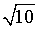but they are still small. Thus, we can do the computations with 1,000 iterations, and perhaps fewer. This is important for the simulations we do next.

Simulation study

The purpose of the simulation study is to investigate the effects of the familial correlation and the disparity between the ignorable and the nonignorable selection models. We have generated data from the nonignorable selection model, and we have fit both the ignorable and the nonignorable selection models. Here we use a single Π and a single θ. We have taken p = .257, Π = .371 and n = 100 to obtain data similar to Crow's data. To study the effect of the familial correlation, we have taken θ = .02, a small value and θ = .20, a larger value.

We have generated 1000 data sets from the nonignorable selection model. From Crow's data, we have obtained the distribution of the ten family sizes 1, 2, . . . , 10. The frequencies of the family sizes are 9, 24, 16, 13, 9, 2, 4, 1, 1, 1. Thus, using the table method, we draw 100 family sizes for each of the 1000 simulated data sets. Now, noting that

p(ak,rk|p, Π, θ)=p(ak|rk, Π)p(rk|p, Π, θ),

We use the composition method to draw rk from p(rk | p, Π, θ), and with this value of rk, we draw ak from p(ak|rk, θ), where p(rk|p, Π, θ) is given in (A.2) of Appendix A, and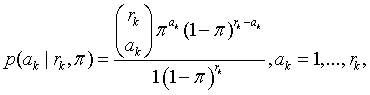a truncated binomial distribution. It is easy to draw ak using a rejection method: draw ak ~Binomial (rk, Π), and take ak whenever it is not 0. We repeat this process for all 100 families.

We have used 1000 iterates to fit each model to the 1000 data sets. For each data set we have computed (a) the posterior mean, posterior standard deviation and the width of the 95% credible interval of each parameter; (b) the probability content of each interval by calculating the proportion of intervals containing the true value of each of the three parameters; and (c) the bias and the mean squared error. In (c) we calculated Abias, which is the average over the 1000 simulations of the absolute deviations of the posterior mean from the true value, and APMSE, which is the average over the 1000 simulations of the square of the deviations of the posterior mean from the true value plus posterior variance. We have also presented standard errors of the quantities in (a), (b) and (c).

In Table 3 we present the results for the simulation study. We consider each measure in turn. The posterior means are in order under the nonignorable selection model, but not under the ignorable selection model; the estimates for p and Π are too large (relative to the true values) as the two examples show. The posterior standard deviations are smaller under the ignorable selection model, more than 100% smaller in some cases. This also makes the 95% credible interval much shorter under the ignorable selection model. The probability contents of the 95% credible intervals are not much smaller than the nominal value under the nonignorable selection model; under the ignorable selection model they are virtually 0 except at θ = .02, where it is really too large. The Abias and APMSE are much smaller under the nonignorable selection model.

θ Model Par PM PSD W C Abias APMSE
.02 Nig p .254.0011 .030.0001 .116.0003 .924.0084 .027.0006 .002.0001
π .379.0023 .066.0002 .254.0007 .911.0090 .059.0014 .010.0002
θ .049.0008 .032.0003 .107.0012 .950.0069 .029.0008 .003.0001
Ig p .441.0008 .026.0000 .101.0001 .000.0000 .184.0008 .035.0003
π .709.0011 .035.0000 .137.0002 .000.0000 .338.0011 .117.0007
θ .017.0002 .015.0001 .045.0003 1.000.0000 .005.0001 .000.0000
.20 Nig p .259.0012 .034.0001 .129.0003 .917.0087 .029.0007 .003.0001
π .373.0019 .055.0001 .213.0006 .905.0093 .049.0012 .007.0002
θ .206.0019 .055.0002 .210.0006 .924.0084 .048.0011 .007.0001
Ig p .489.0010 .029.0000 .110.0002 .000.0000 .232.0010 .056.0005
π .648.0012 .035.0000 .134.0001 .000.0000 .277.0012 .080.0006
θ .061.0009 .035.0003 .121.0011 .064.0077 .139.0009 .021.0002

Table 3: Simulation study to compare posterior means, posterior standard deviations and 95% credible intervals of the parameters p, ?and ? by model and the true value of ?

Therefore, the ignorable selection model gives badly inaccurate estimates with artificially high precision. Under the nonignorable selection model the point and interval estimates are acceptable, but not those for the ignorable selection model. In fact, Abias and APMSE favor the nonignorable selection model. There is some effect of the intra-class correlation.

Heterogeneous Probabilities and Correlations

We generalize the discussion in this paper by considering heterogeneous proband probabilities and familial correlations. Specifically, in heterogeneous proband probabilities section, we consider the case in which there are different proband probabilities, and in heterogeneous familial correlations section, we consider the case in which there are different familial correlations.

Heterogeneous proband probabilities

Here we allow the proband probabilities to vary with the number of affected siblings within each family. Crow's data have four values (1, 2, 3, 4) for the number affected. So for Crow's data there are four different parameters (Π1,..., Π4). Thus, generally let Πrk denote the proband probabilities, and d be the number of distinct proband probabilities (Π1,..., Πd).

Then, with this simple adjustment the likelihood function for the n families is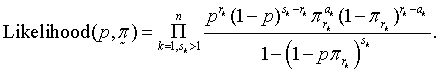(9)

A priori we assume thatUniform (0,1). Then, the joint posterior density for (p, Π) is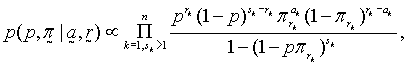(10)

0 < p, Π1,..., Πd< 1. To make posterior inference about (p, Π), it is more convenient to use the griddy Gibbs sampler .

The griddy Gibbs sampler is performed as follows. We obtain the conditional posterior distribution of each parameter in turn. For p, the conditional posterior density is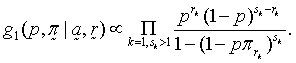(11)

Now, given p, a and r, π t, t = 1, . . . , d, are independent with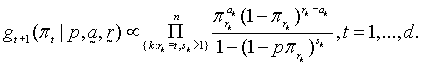(12)

Using a grid, we draw a random variate from (11), and with this value of p we have drawn independently the d remaining parameters from (12). Actually, we started with p = .35, and we drew from (12) first and (11) second; this is useful because we only need to specify one starting value of p. Again, we use 100 grid intervals for each conditional. Conservatively, we "burn in" 1000 iterates, and we use the next 10,000 values to make posterior inference about . The griddy Gibbs sampler settles down very quickly, and there are virtuallyno auto correlations in the iterates. We use these iterates to do inference as in the standard Monte Carlo procedure.

For Crow's data, the posterior mean, posterior standard deviation and 95% credible interval for p are .294, .036, (.229, .369); the numerical standard error is .00035. Here, the hypothesis of an autosomal recessive is not in dispute, but we note that the 95% credible interval moves over a little to the right. Compare the posterior mean of p of .268 with a single proband probability versus .294 with five proband probabilities. In Table 4 we present posterior inference about the proband probabilities. We can see that the parameters are different, and there are for all practical purposes only two distinct values of Π (i.e., when variability is taken into consideration, the last three proband parameters may be taken to be equal). Thus, we repeat the computations with just two distinct values of Π. Now, the posterior mean, posterior standard deviation and 95% credible interval for p are .293, .037, (.221, .361); the numerical standard error is .00039. The 95% credible intervals for the two values of Π are (.732, 1.000) and (.181, .457). Again posterior inference about p does not seem to be sensitive to the number of Π's used, when more than one proband probability is used.

 PM PSD NSE Interval a.Singleprobandprobability p .271 .034 .0003 (.206, .340) π .364 .078 .0008 (.217,.521) b.Nocollapsing P .294 .036 .0003 (.229, .369) π 1 .911 .091 .0010 (.732,1.000) π 2 .314 .097 .0010 (.129,.502) π 3 .372 .108 .0011 (.165,.578) π 4 .332 .177 .0017 (.000,.577) c.Collapsing P .293 .036 .0004 (.221, .361) π 1 .911 .090 .0010 (.732,1.000) π 2 .314 .072 .0007 (.181,.457)

Table 4: Posterior mean (PM), posterior standard deviation (PSD), numerical standard error (NSE) and 95% credible interval for the segregation parameter and the proband probabilities for Crow’s data.

In Figure 1 we have presented the empirical distributions of p under the three scenarios. The empirical posterior density of p is different from the posterior densities of p corresponding to five proband probabilities and the five proband probabilities collapsed into just two distinct proband probabilities; these latter two empirical posterior densities are similar.

Figure 1: Plots of the posterior density estimators of the segregation ratios by number of proband parameters (one - p1, different - p2, collapsed - p3) for Crow’s data.

Heterogeneous familial correlations

We now allow the intra-class correlation to vary with family size sk. Thus, the intra-class correlations are θ sk, k = 1, . . . , n. For Crow's data there are 10 different family sizes, so there are 10 distinct correlation parameters θ1,..., θ10 . In general, we assume that there are g parameters. Note that for a one-sibling family, θ1 = 0. Again, we take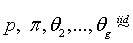uniform (0, 1). Then, the joint posterior density is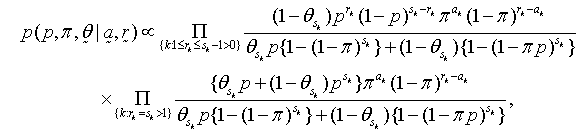(13)

0 <p, π, θ2,..., θg < 1.

Again, we use the griddy Gibbs sampler  to perform the computation. We performgrids on each of the conditional posterior densities which do not have simple forms as can be easily seen from (13). [Looking at (13) the three conditional posterior densities,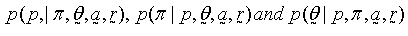can be easily written down]. We "burn in"1000 iterates, and we use the next 10,000 to make posterior inference. The autocorrelationswere negligible for all parameters, and there were fast convergence as is evident in the quick settling down of the trace plots.

In Table 5 we present results corresponding to different intra-class correlations. With nine intra-class correlations, the posterior mean, posterior standard deviation and the 95%credible intervals of p are .259, .033 and (.200, .329). The credible interval moves over a little to the left. The nine intra-class correlations are all small, but partitioning according to the intra-class correlations, one can see two groups with sibship sizes 2, 8, 10 and the other with sibship sizes 3, 4, 5, 6, 7, 9. So we collapsed the nine different correlations to two distinct ones. As expected, there are some changes in the standard errors and intervals, but these are small.

 PM PSD NSE Interval a. SingleFamilialCorrelation p .257 .033 .0003 (.190, .320) π .371 .079 .0008 (.217,.520) θ .026 .024 .0002 (.000, .074) b. Nocollapsing p .259 .033 .0003 (.200, .329) π .372 .079 .0008 (.221, .527) θ2 .006 .005 .0001 (.000, .010) θ3 .027 .016 .0002 (.010, .058) θ4 .029 .018 .0002 (.010, .065) θ5 .030 .020 .0002 (.010, .069) θ6 .032 .021 .0002 (.010, .074) θ7 .034 .023 .0002 (.010, .079) θ8 .020 .022 .0002 (.000, .065) θ9 .037 .026 .0002 (.010, .090) θ10 .028 .027 .0002 (.000, .084) c. Collapsing p ..258 .033 .0003 (.190, .320) π .371 .079 .0008 (.217,.520) θ2 .028 .027 .0002 (.000, .084) θ3 .026 .024 .0002 (.000, .074)

Table 5: Posterior mean (PM), posterior standard deviation (PSD), numerical standard error (NSE) and 95% credible interval for p, Π, θk, k = 2, . . . , 10 for Crow’s data.

#### Conclusion

Concluding remarks

When one wants to find out about the proportion of people with a rare disease, one cannot take a random sample from the population. It is convenient to take a random sample of the cases that appear. Thus, clearly this sample is biased (i.e., there is a selection bias). An important example in genetics occurs when one is interested in the segregation ratio for a rare recessive disease. This problem exists over a century, and there are many solutions depending on the sampling scheme. The Bayesian solutions have some merit though.

We have considered the problem of estimating the segregation parameters and the proband probabilities when there is an autosomal recessive disease. We make three useful contributions which are (a) we provide a full Bayesian analogue to the available non-Bayesian solutions; (b) we extend the methodology to reflect an intra-correlation within family; (c) we discuss the cases when there are heterogeneous proband probabilities and familial correlations. The computation in (a) and (b) is easy because we can use Monte Carlo methods with only random samples. However, in (c) we used the griddy Gibbs sampler.

In this paper we have not reported on the ascertainment bias that occurs in single nucleotide polymorphism (SNP) discovery. This is an enormously important problem with implications for the study of many genetic disorders. Our work on rare autosomal recessive disorders is a preamble to the study of ascertainment bias in SNP discovery. However, we give a brief description.

Ascertainment bias in SNP discovery

In SNP discovery, one can measure the polymorphism at the ith nucleotide throughout the population by using allele frequency. Other measures that are potentially more useful are heterozygosity (H) and the polymorphism information content (PIC) . For s individuals in the panel, let ci be the number of ones among the 2s zeros and ones at the ith nucleotide, let di denote either H or PIC at the ith nucleotide. Then,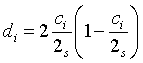for H, and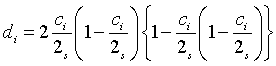for PIC. [Note that the number of individuals at eachnucleotide is the same fixed number s, and there are 2s zeros and ones.] Then, analogous to probability proportion to size in survey sampling, one can take Πi∝di, i = 1, . . . , N, for the N nucleotides with n sampled. Here, it is not really the individuals that are sampled, but n nucleotides are ascertained out of N ≈ 106. Now, letting Ii=1 if the ith nucleotide is selected, and Ii= 0 if the ith nucleotide is not selected, then under Poisson (Bernoulli) sampling,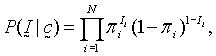(14)

where the proband probabilities are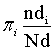where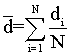Note that the di are observed for the nucleotides in the panel. In Poisson sampling the assumption (14) is reasonable. Then, a reasonable assumption is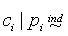Binomial(2s,pi), i = 1,…,N.                                                                                    (15)

Both assumptions (14) and (15) are the basis of a model for SNP discovery under ascertainment bias. All structures and quantities of interest can be added as are needed. Different correlation structures among the nucleotides can be specified. The important disease-causing genes can be assessed, and more accurate results from case-control studies, used in SNP discovery, can be obtained.

#### References

Select your language of interest to view the total content in your interested language

### Article Usage

• Total views: 12101
• [From(publication date):
March-2011 - Jul 18, 2019]
• Breakdown by view type
• HTML page views : 8297Can't read the image? click here to refresh